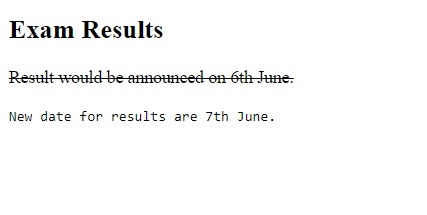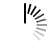HTML Computer Code Elements

To display computer codes on the web page, some elements are provided in HTML. Let us see those elements one by one −

• <kbd>
• <pre>
• <code>
• <var>
• <samp>

<kbd> element in HTML

The <kbd> tag is used to define the keyboard input. Use this when you want the user to type on their keyboard, for example, shortcut keys Ctrl+C for copy, Esc for exit, etc.

Let us now see an example to implement the <kbd> tag −

Example

Live Demo

```<!DOCTYPE html>
<html>
<body>
<h2>Shortcut Keys</h2>
<p>Use the following shortcut keys −</p>
<p><strong>Cut</strong> − <kbd>CTRL</kbd>+<KBD>X</kbd></p>
<p><strong>Copy</strong> − <kbd>CTRL</kbd>+<KBD>C</kbd></p>
<p><strong>Paste</strong> − <kbd>CTRL</kbd>+<KBD>V</kbd></p>
<p><strong>Undo</strong> − <kbd>CTRL</kbd>+<KBD>Z</kbd></p>
</body>
</html>```

Output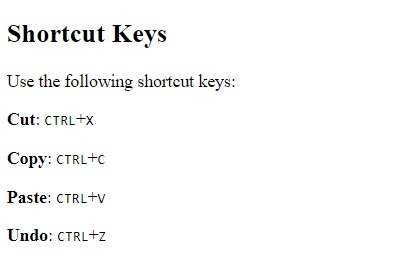<pre> element in HTML

The <pre> tag in HTML is used to set preformatted text. The text in it preserved spaces and line breaks i.e. the text appears the same in the web page as it is added under the HTML code. Let us now see an example to implement the <pre> tag −

Example

Live Demo

```<!DOCTYPE html>
<html>
<body>
<pre>
This is a demo text
and will appear
in the same format as
it
is visible
here. The pre tag displays
the text in a fixed-width
font. It preserves
both spaces and
line breaks as you can see
here.
</pre>
</body>
</html>```

Output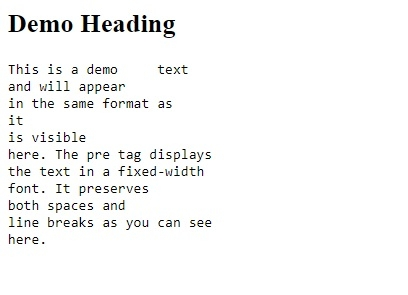<code> element in HTML

The <code> tag is used to format code in an HTML document. For example, if you want to write Java code and format it properly, then use the <code> element in HTML. Let us now see an example to implement the <code> element in HTML −

Example

Live Demo

```<!DOCTYPE html>
<html>
<body>
<h2>C++</h2>
<code>
#include <iostream>
</code>
<h2>C</h2>
<code>
#include <stdio>
</code>
</body>
</html>```

Output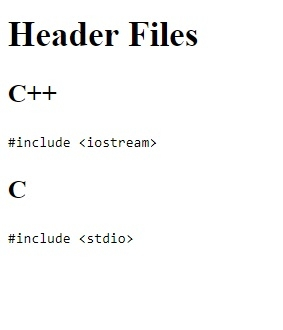<var> element in HTML

The <var> element in HTML is used to display mathematical expression for calculations.

Let us now see an example to implement the <var> tag in HTML −

Example

Live Demo

```<!DOCTYPE html>
<html>
<body>
<h2>Mathematical Equation</h2>
Sample equation − <var>2x</var> - <var>2z</var> = <var>3y</var> + 9
</body>
</html>```

Output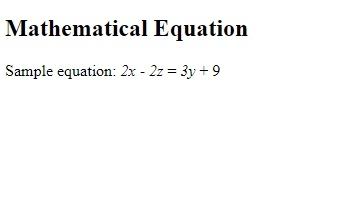<samp> element in HTML

The <samp> tag in HTML is a phrase tag like <em>, <code>, <strong>, etc and is used to format text in an HTML document.

Let us now see an example to implement the <samp> element −

Example

Live Demo

```<!DOCTYPE html>
<html>
<body>
<h2>Exam Results</h2>
<p><s>Result would be announced on 6th June.</s></p>
<samp>New date for results are 7th June.</samp>
</body>
</html>```

Output